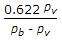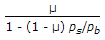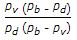# Mechanical Engineering - Heat Transfer, Refrigeration and Air Conditioning

### Exercise :: Heat Transfer, Refrigeration and Air Conditioning - Section 6

16.

A Bell Coleman refrigerator working on dense air system as compared to open air system, for the same range of temperature, results in higher coefficient of performance.

 A. Agree B. Disagree

Explanation:

No answer description available for this question. Let us discuss.

17.

The ratio of surface convection resistance to the internal conduction resistance is known as

 A. Grashoff number B. Biot number C. Stanton number D. Prandtl number

Explanation:

No answer description available for this question. Let us discuss.

18.

Relative humidity is given by (where pv = Partial pressure of water vapour, pb = Barometric pressure, ps = Pressure of saturated air, pd = Pressure at dry bulb temperature, pw = Saturation pressure corresponding to wet bulb temperature, and μ = Degree of saturation)

 A.B.C.D. none of these

Explanation:

No answer description available for this question. Let us discuss.

19.

Kirchhoff's law states that

 A. the total radiation from a black body per second per unit area is directly proportional to the fourth power of the absolute temperature B. the wave length corresponding to the maximum energy is proportional to the absolute temperature C. the ratio of the emissive power and absorptive power of all bodies is the same and is equal to the emissive power of a perfectly black body D. none of the above

Explanation:

No answer description available for this question. Let us discuss.

20.

Dew point temperature is the temperature of air recorded by a thermometer, when the moisture present in it begins to condense.

 A. Correct B. Incorrect